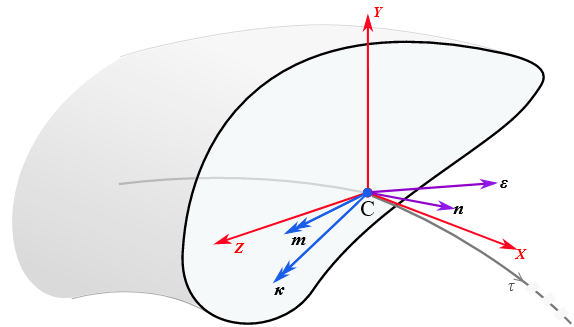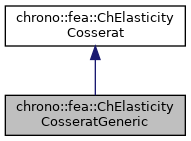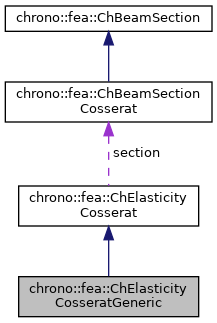chrono::fea::ChElasticityCosseratGeneric Class Reference

## Description

Generic linear elasticity for a Cosserat beam using directly a 6x6 matrix [E] as user-input data.

The [E] matrix values ("rigidity" values) can be computed from a preprocessing stage using a FEA analysis over a detailed 3D model of a chunk of beam, hence recovering the 6x6 matrix that connects yxz displacements "e" and xyz rotations "k" to the xyz cut-force "n" and xyz cut-torque "m" as in $$(m,n)=[E](e,k)$$ where $$e, k, m, n$$ are expressed in the centerline reference. Using a matrix of rigidity values, the model does not assume homogeneous elasticity and bypasses the need of entering E or G values. This can be shared between multiple beams.#include <ChBeamSectionCosserat.h>

Inheritance diagram for chrono::fea::ChElasticityCosseratGeneric:[legend]
Collaboration diagram for chrono::fea::ChElasticityCosseratGeneric:[legend]

## Public Member Functions

ChMatrixNM< double, 6, 6 > & Ematrix ()
Access the E matrix, for getting/setting its values. More...

virtual void ComputeStress (ChVector<> &stress_n, ChVector<> &stress_m, const ChVector<> &strain_e, const ChVector<> &strain_k) override
Compute the generalized cut force and cut torque. More...

virtual void ComputeStiffnessMatrix (ChMatrixNM< double, 6, 6 > &K, const ChVector<> &strain_e, const ChVector<> &strain_k) override
Compute the 6x6 tangent material stiffness matrix [Km] = dσ/dε. More...Public Attributes inherited from chrono::fea::ChElasticityCosserat
ChBeamSectionCosseratsection

## ◆ ComputeStiffnessMatrix()

 void chrono::fea::ChElasticityCosseratGeneric::ComputeStiffnessMatrix ( ChMatrixNM< double, 6, 6 > & K, const ChVector<> & strain_e, const ChVector<> & strain_k )
overridevirtual

Compute the 6x6 tangent material stiffness matrix [Km] = dσ/dε.

Parameters
 K 6x6 stiffness matrix strain_e local strain (deformation part): x= elongation, y and z are shear strain_k local strain (curvature part), x= torsion, y and z are line curvatures

Reimplemented from chrono::fea::ChElasticityCosserat.

## ◆ ComputeStress()

 void chrono::fea::ChElasticityCosseratGeneric::ComputeStress ( ChVector<> & stress_n, ChVector<> & stress_m, const ChVector<> & strain_e, const ChVector<> & strain_k )
overridevirtual

Compute the generalized cut force and cut torque.

Parameters
 stress_n local stress (generalized force), x component = traction along beam stress_m local stress (generalized torque), x component = torsion torque along beam strain_e local strain (deformation part): x= elongation, y and z are shear strain_k local strain (curvature part), x= torsion, y and z are line curvatures

Implements chrono::fea::ChElasticityCosserat.

## ◆ Ematrix()

 ChMatrixNM& chrono::fea::ChElasticityCosseratGeneric::Ematrix ( )
inline

Access the E matrix, for getting/setting its values.

This is the matrix that defines the linear elastic constitutive model as it maps yxz displacements "e" and xyz rotations "k" to the xyz cut-force "n" and xyz cut-torque "m" as in $$(m,n)=[E](e,k)$$

The documentation for this class was generated from the following files:
• /builds/uwsbel/chrono/src/chrono/fea/ChBeamSectionCosserat.h
• /builds/uwsbel/chrono/src/chrono/fea/ChBeamSectionCosserat.cpp